Next: Other comments, examples and Up: Bayesian unfolding Previous: Bayes' theorem stated in   Contents

# Unfolding an experimental distribution

If one observes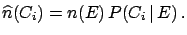events with effect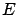, the expected number of events assignable to each of the causes is(7.2)

As the outcome of a measurement one has several possible effects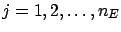(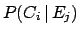) for a given cause. For each of them the Bayes formula () holds, and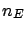can be evaluated. Let us write () again in the case of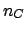possible effects7.1, indicating the initial probability of the causes with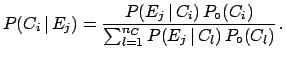: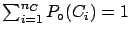(7.3)

One should note the following.
•, as usual. Note that if the probability of a cause is initially set to zero it can never change, i.e. if a cause does not exist it cannot be invented.
•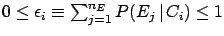. This normalization condition, mathematically trivial since it comes directly from (), indicates that each effect must come from one or more of the causes under examination. This means that if the observables also contain a non-negligible amount of background, this needs to be included among the causes.
•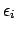. There is no need for each cause to produce at least one of the effects.gives the efficiency of finding the causein any of the possible effects.

Afterexperimental observations one obtains a distribution of frequencies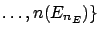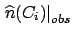. The expected number of events to be assigned to each of the causes (taking into account only the observed events) can be calculated by applying () to each effect: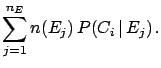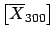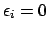(7.4)

When inefficiency7.2 is also brought into the picture, the best estimate of the true number of events becomes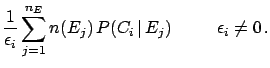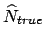(7.5)

From these unfolded events we can estimate the true total number of events, the final probabilities of the causes and the overall efficiency: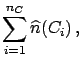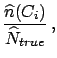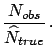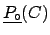If the initial distribution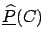is not consistent with the data, it will not agree with the final distribution. The closer the initial distribution is to the true distribution, the better the agreement is. For simulated data one can easily verify that the distributionlies betweenand the true one. This suggests proceeding iteratively. Fig.shows an example of a bidimensional distribution unfolding.

More details about iteration strategy, evaluation of uncertainty, etc. can be found in Ref. .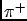I would just like to comment on an obvious criticism that may be made: the iterative procedure is against the Bayesian spirit, since the same data are used many times for the same inference''. In principle the objection is valid, but in practice this technique is a trick'' to give to the experimental data a weight (an importance) larger than that of the priors. A more rigorous procedure which took into account uncertainties and correlations of the initial distribution would have been much more complicated. An attempt of this kind can be found in Ref. . Examples of unfolding procedures performed with non-Bayesian methods are described in Refs.  and .

Note added: A recent book by Cowan contains an interesting chapter on unfolding.Next: Other comments, examples and Up: Bayesian unfolding Previous: Bayes' theorem stated in   Contents
Giulio D'Agostini 2003-05-15